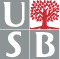# MAT 311 Number Theory

In addition to homework you will be required to hand in a research/scholarship/computing projects. Projects with a nontrivial writing component may be used to satisfy the Mathematics Upper Division Writing Requirement. The project should be handed in final form by 04/17. You need to make your selection and also inform me of it (in writing) by 02/28. Here is a list of suggestions for your projects:

1. Discuss the existence, number, etc of non-negative solutions of a diophantine equation ax+by=n where a,b,n are positive, (a,b)=1. For instance
• Show that whenever n≥(a-1)(b-1) then there always are nonnegative solutions.
• Show that if n=(a-1)(b-1)-1 then there are no nonnegative solutions.
• For how many nonnegative integers n<(a-1)(b-1)-1 does there exist a non-negative solution to the above equation?
• Write a program to find all n for which the above equation has nonnegative solutions.
2. Give estimates for the number of bit operations needed to compute each of the following:
• n!
• n choose k
• Find the binary expansion of a number given its decimal expansion.
(Hint: Read more in Rosen Section 2.3)
3. Write a program to explore the following statements. For those you believe true try to provide a proof!
• If p1, p2, ... ,pt are primes not exceeding n then p1p2...pt≤4n.
• The n-th prime number pn is smaller than 22n-1.
• The n-th prime number pn is smaller or equal than 2n.
4. Fermat numbers are numbers of the form Fn=22n+1, for positive integers n.
• Find the last 2 digits of a Fermat number Fn in its decimal expansion.
• Estimate the number of decimal digits in the Fermat number Fn.
• Show that (Fn, Fm)=1, for distinct positive integers n and m.
• What is the the gcd of Fn and n?
5. Write a program that finds all twin primes less than 20,000. Hardy and Littlewood conjectured that the number of twin primes not exceeding n is asymptotic to C n/(ln n)2 for a constant C, approximately equal to 0.66016. Determine how accurate this asymptotic formula is for values of n as large as you can compute.
6. Prove that unique factorization holds in Z+Z[i] (where i is the imaginary unit). Describe an analogue of the Euclidean algorithm in this context. What happens for numbers of type Z+Z√5?
7. Show that if p is a prime of the form 4k+3 and q=2p+1 is prime, then q divides the Mersenne number Mp=2p-1. (Hint: use Legendre symbols).
8. Derive an explicit formula that gives the day of the week of any day of any given year in the Julian or the Gregorian calendars. Use these formulas to find the day of the week of the following important dates (Hint: Use the Julian calendar before September 3, 1752 and the Gregorian calendar after that date):
• October 12, 1492 (Columbus sights land in the Caribbean)
• July 4, 1776 (US Declaration of Independence)
• July 20, 1969 (First man on the moon)
Which of your birthdays, until your one hundredth, fall on the same day of the week as the day you were born?
9. A Carmichael number is an absolute pseudoprime n, that is a number n such that an-1≅1 (mod n) for all positive integers a such that (a,n)=1.
• Show that if n is a Carmichael number then it is squarefree.
• Show that if n=p1p2...pn is a product of distinct primes such that pi-1|n-1 for all i, then n is a Carmichael number. Use this to show that 564651361 is a Carmichael number. Can you write a program to find other Carmichael numbers?
• Find as many Charmichael numbers of the form (6m+1)(12m+1)(18m+1) as you can.
10. An integer n is called highly composite if τ(n)= τ(m) for all integers m<n. Find all highly composite numbers not exceeding 10,000.
11. Suppose that a new Stony Brook ID will be implemented where each student gets assigned a 10 digit code word x1 x2...x10. Each digit is a decimal digit, and the valid codes are those satisfying the congruences ∑xi ≅ ∑ixi ≅ ∑i2xi ≅ ∑i3xi ≅ 0 (mod 11).
• How many valid 10 digit code words there are? Will there be enough many such IDs for all the SB students?
• Show how any two errors in such a code word can be corrected.
• For instance suppose we have typed 0204906710 as a code word but we have made two mistakes. What should have been the correct code word?
12. Discuss the cryptanalysis of Vigenere ciphers and write a program to decrypt messages which have been encrypted using such ciphers.

For any of the programming projects, please email me at sorin at math.sunysb.edu both the program source code (in readable form -- indented and commented) and the project, and please hand in the program outline and a reasonable amount of program output. You can use any programming language you like (within reasonable limits - i.e., a language for which there exist easily available compilers). Preferred ones are Maple, Mathematica (yes, they are programming languages), C, OCAML and Java, but you can also use C++, Pascal, Python, Fortran, Lisp, Turing machine...